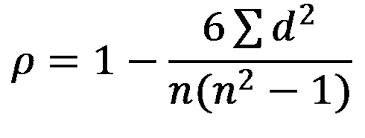# Tag Archives: spearman# Spearman Rank Correlation

Spearman rank correlation aka ρ is used to measure the strength of the relationship between two variables. You may be already wondering what is the difference between Spearman rank correlation and Person product moment correlation. The difference is that Spearman rank correlation is a non-parametric test while Person product moment correlation is a parametric test.

A non-parametric test does not have to comply with the assumptions ofparametric test such as the data being normally distributed. This allows a researcher to still make inferences from data that may not have normality. In addition, non-parametric test are used for data that is at the ordinal or nominal level. In many ways, Spearman correlation and Pearson product moment correlation compliment each other. One is used in non-parametric statistics and the other for parametric statistics and each analyzes the relationship between variables.

If you get suspicious results from your Pearson product moment correlation analysis or your data lacks normality Spearman rank correlation may be useful for you if you still want to determine if there is a relationship between the variables. Spearmen correlation works by ranking the data within each variable. Next, the Pearson product moment correlation is calculated between the two sets of rank variables. Below are the assumptions of Spearman correlation test.

• Subjects are randomly selected
• Observations are at the ordinal level at least

Below are the steps of Spearman correlation

1. Setup the hypotheses
1. H0: There is no correlation between the variables
2. H1: There is a correlation between the variables
2. Set the level of significance
3. Calculate the degrees of freedom and find the t-critical value (computer does this for you)
4. Calculate the value of Spearman correlation or ρ (computer does this for you)
5. Calculate the t-value(computer does this for you) and make a statistical decision
6. State conclusion

Here is an example

A clerk wants to see if there is a correlation between the overall grade students get on an exam and  the number of words they wrote for their essay. Below are the results

1                             79                           147
2                             76                           143
3                             78                           147
4                             84                           168
5                             90                           206
6                             83                           155
7                             93                           192
8                             94                           211
9                             97                           209
10                           85                           187
11                           88                           200
12                           82                           150

Note: The computer will rank the data of each variable with a rank of 1 being the highest value of a variable and a rank 12 being the lowest value of a variable. Remember that the computer does this for you.

Step 1: State hypotheses
H0: There is no relationship between grades and words on the essay
H1: There is a relationship between grades and words on the essay

Step 2: Determine level of significance
Level set to 0.05

Step 3: Determine critical t-value
t = + 2.228 (computer does this for you)

Step 4: Compute Spearman correlation
ρ = 0.97 (computer does this for you)
Note: This correlation is very strong. Remember the strongest relationship possible is + 1

Step 5: Calculate t-value and make a decision
t = 12.62   ( the computer does this for you)
Since the computed t-value of 12.62 is greater than the t-critical value of 2.228 we reject the null hypothesis

Step 6: Conclusion
Since the null hypotheses are rejected, we can conclude that there is evidence that there is a strong relationship between exam grade and the number of words written on an essay. This means that a teacher could tell students they should write longer essays if they want a higher grade on exams